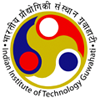•Department of Electronics and Electrical Engineering
Indian Institute of Technology GuwahatiDepartment of Electronics
and Electrical Engineering

### Probability and Stochastic Processes

Course Contents:

Axiomatic definitions of probability; conditional probability, independence and Bayes theorem, continuity property of probabilities, Borel-Cantelli Lemma; random variable: probability distribution, density and mass functions, functions of a random variable; expectation, characteristic and moment-generating functions; Chebyshev, Markov and Chernoff bounds; jointly distributed random variables: joint distribution and density functions, joint moments, conditional distributions and expectations, functions of random variables; random vector- mean vector and covariance matrix, Gaussian random vectors; sequence of random variables: almost sure and mean-square convergences, convergences in probability and in distribution, laws of large numbers, central limit theorem; random process: probabilistic structure of a random process; mean, autocorrelation and autocovariance functions; stationarity - strict- sense stationary and wide-sense stationary (WSS) processes: time averages and ergodicity; spectral representation of a real WSS process-power spectral density, cross-power spectral density, linear time-invariant systems with WSS process as an input- time and frequency domain analyses; examples of random processes: white noise, Gaussian, Poisson and Markov processes.

Texts / References:

1. H. Stark and J. W. Woods, Probability and Random Processes with Applications to Signal Processing, Prentice Hall, 2002.
2. A. Papoulis and S. U. Pillai, Probability, Random Variables and Stochastic Processes, 4th Edn., McGraw-Hill, 2002.
3. B. Hajek, An Exploration of Random Processes for Engineers, ECE534 Course Notes, 2011. http://www.ifp.illinois.edu/~hajek/Papers/randomprocesses.html